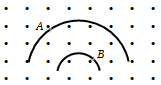An electron and a proton enter a magnetic field perpendicularly. Both have the same kinetic energy. Which of the following is true:

1. Trajectory of electron is less curved

2. Trajectory of proton is less curved

3. Both trajectories are equally curved

4. Both move on a straight-line path

Concept Questions :-

Lorentz force
High Yielding Test Series + Question Bank - NEET 2020

Difficulty Level:

A proton, a deuteron, and an $\alpha -$ particle having the same kinetic energy are moving in circular trajectories in a constant magnetic field. If ${r}_{p},{r}_{d}$ and ${r}_{\alpha }$ denote respectively the radii of the trajectories of these particles, then:

1. ${r}_{\alpha }={r}_{p}<{r}_{d}$

2.  ${r}_{a}>{r}_{d}>{r}_{p}$

3. ${r}_{\alpha }={r}_{d}>{r}_{p}$

4.  ${r}_{p}={r}_{d}={r}_{\alpha }$

Concept Questions :-

Lorentz force
High Yielding Test Series + Question Bank - NEET 2020

Difficulty Level:

An electron and a proton with equal momentum enter perpendicularly into a uniform magnetic field, then :

(a) The path of proton shall be more curved than that of electron

(b) The path of proton shall be less curved than that of electron

(c) Both are equally curved

(d) Path of both will be a straight line

Concept Questions :-

Lorentz force
High Yielding Test Series + Question Bank - NEET 2020

Difficulty Level:

One proton beam enters a magnetic field of ${10}^{-4}T$ normally, Specific charge = ${10}^{11}C/kg.$ velocity = ${10}^{7}m/s.$ What is the radius of the circle described by it:

1. $0.1m$

2. $1m$

3. $10m$

4. None of these

Concept Questions :-

Lorentz force
High Yielding Test Series + Question Bank - NEET 2020

Difficulty Level:

In a cyclotron, the angular frequency of a charged particle is independent of :

1. Mass

2.  Speed

3. Charge

4. Magnetic field

Concept Questions :-

Cyclotron
High Yielding Test Series + Question Bank - NEET 2020

Difficulty Level:

Maximum kinetic energy of the positive ion in the cyclotron is :

(a) $\frac{{q}^{2}B{r}_{0}}{2m}$                         (b) $\frac{q{B}^{2}{r}_{0}}{2m}$

(c) $\frac{{q}^{2}{B}^{2}{r}_{0}^{2}}{2m}$                         (d) $\frac{qB{r}_{0}}{2{m}^{2}}$

Concept Questions :-

Cyclotron
High Yielding Test Series + Question Bank - NEET 2020

Difficulty Level:

An electron of mass m and charge q is traveling with a speed v along a circular path of radius r at right angles to a uniform of the magnetic field B. If the speed of the electron is doubled and the magnetic field is halved, then resulting path would have a radius of:

1. $\frac{r}{4}$

2. $\frac{r}{2}$

3. $2r$

4. $4r$

Concept Questions :-

Lorentz force
High Yielding Test Series + Question Bank - NEET 2020

Difficulty Level:

Cyclotron cannot be used to accelerate

(a) Electrons                        (b) Neutrons

(c) Positive ions                    (d) Both (1) and (2)

Concept Questions :-

Cyclotron

Difficulty Level:

Two particles A and B of masses ${m}_{A}$ and ${m}_{B}$ respectively and having the same type of charge are moving in a plane. A uniform magnetic field exists perpendicular to this plane. The speeds of the particles are ${v}_{A}$ and ${v}_{B}$ respectively, and the trajectories are as shown in the figure. Then(a) ${m}_{A}{v}_{A}<{m}_{B}{v}_{B}$                               (b) ${m}_{A}{v}_{A}>{m}_{B}{v}_{B}$

(c)                    (d)

Concept Questions :-

Lorentz force
High Yielding Test Series + Question Bank - NEET 2020

Difficulty Level:

Magnetic field due to a ring having n turns at a distance x on its axis is proportional to (if r = radius of ring) :

(a)  $\frac{\mathrm{r}}{\left({\mathrm{x}}^{2}+{\mathrm{r}}^{2}\right)}$                                     (b)  $\frac{{\mathrm{r}}^{2}}{\left({\mathrm{x}}^{2}+{\mathrm{r}}^{2}{\right)}^{3/2}}$

(c)  $\frac{{\mathrm{nr}}^{2}}{\left({\mathrm{x}}^{2}+{\mathrm{r}}^{2}{\right)}^{3/2}}$                                 (d)  $\frac{{\mathrm{n}}^{2}{\mathrm{r}}^{2}}{\left({\mathrm{x}}^{2}+{\mathrm{r}}^{2}{\right)}^{3/{2}^{}}}$

Concept Questions :-

Magnetic field due to various cases